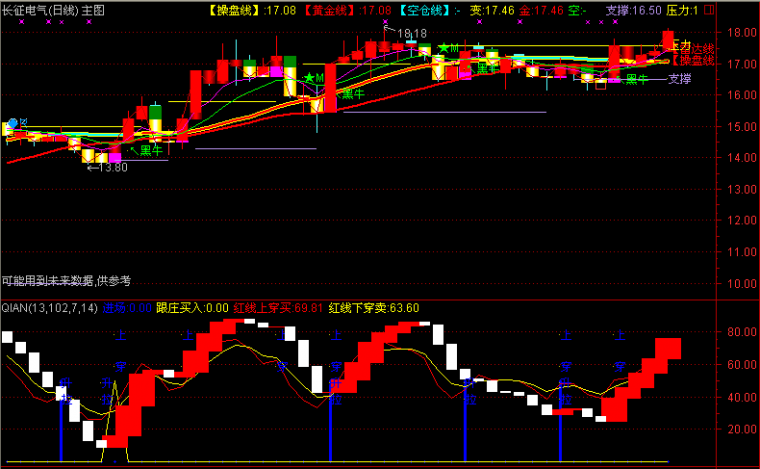﻿ 波段底副图指标公式公式源码-通达信公式 －程序化交易（CXH99.COM）

波段底副图指标公式公式源码[通达信公式]

VARQA赋值:收盘价的20日简单移动平均
RSV赋值:(收盘价-32日内最低价的最低值)/(32日内最高价的最高值-32日内最低价的最低值)
K赋值:RSV的5日[1日权重]移动平均
D赋值:K的3日[1日权重]移动平均
D2赋值:K的2日[1日权重]移动平均
D3赋值:((3)*(K)-(2)*(D))*(99)
QZS赋值:(收盘价的10日指数移动平均+收盘价的32日指数移动平均)/2
VAR1赋值:(收盘价的30日指数移动平均+收盘价的72日指数移动平均)/2

VARQA:=MA(CLOSE,20);
RSV:=(CLOSE-LLV(LOW,32))/(HHV(HIGH,32)-LLV(LOW,32));
K:=SMA(RSV,5,1);
D:=SMA(K,3,1);
D2:=SMA(K,2,1);
D3:=((3)*(K)-(2)*(D))*(99);
QZS:=(EMA(CLOSE,10)+EMA(CLOSE,32))/2;
VAR1:=(EMA(CLOSE,30)+EMA(CLOSE,72))/2;

DRAWICON(CROSS(启动,参考线),参考线,1);(注：由于人数限制，QQ或微信请选择方便的一个联系我们就行，加好友时请简单备注下您的需求，否则无法通过。谢谢您！)

【字体： 】【打印文章】【查看评论

没有相关内容